## UEFA CL round of 16: probabilities table before the draw

Written on 8 December 2017, 12:03am

Tagged with: , ,

A post where football meets science again 🙂 This time, it’s about probabilities.
On 11 December 2017 the UEFA Champions League draw will take place. There will be 16 teams which will be drawn one against each other. There are some restrictions:
– 8 teams are seeded, the other 8 are unseeded. A seeded team can only be drawn against an unseeded team
– teams from the same country cannot be drawn against each other
– teams that already met in the previous round cannot be drawn against each other
Based on these elements, I wanted to calculate the associated probabilities, or other words to reveal the question marks in the matrix below: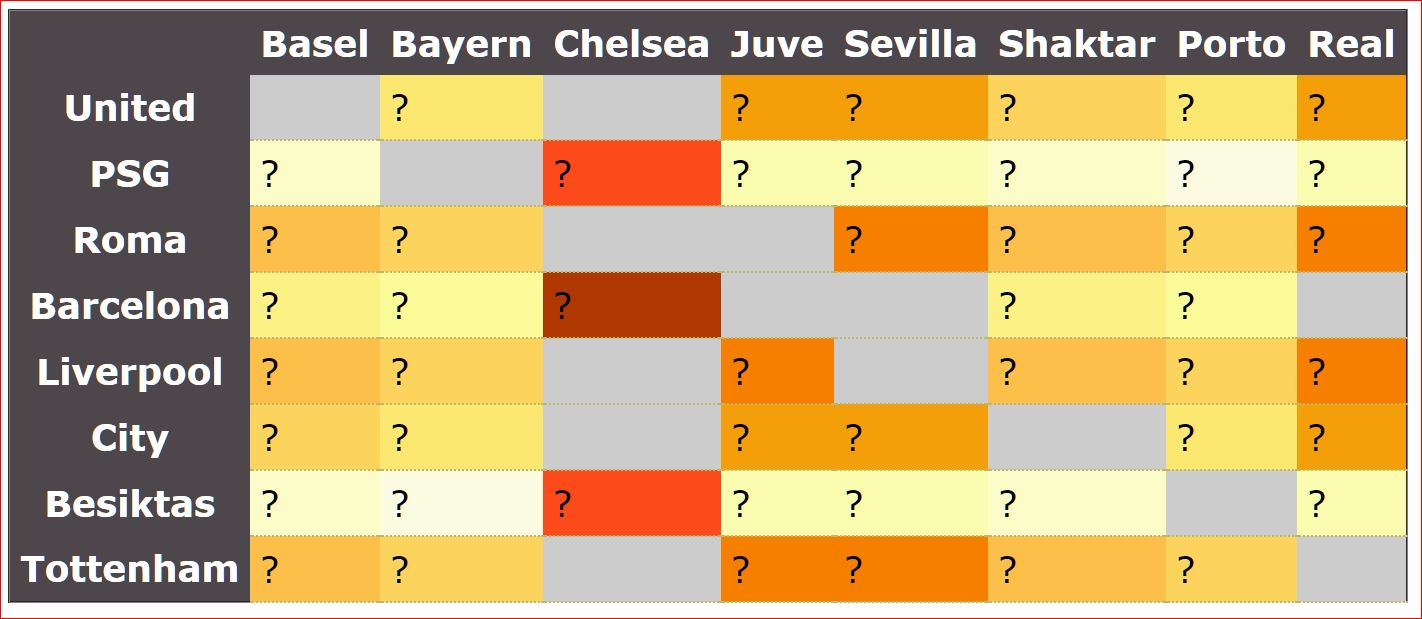(first column – seeded teams, first line – unseeded teams, greyed cells – teams cannot be drawn).

## Try 1: Thursday night

I make a quick PHP script to calculate all the possible permutations (`8!=40320`), then I eliminate the invalid options and find that only `4238` permutations are possible. I count all the possible team pairings as below: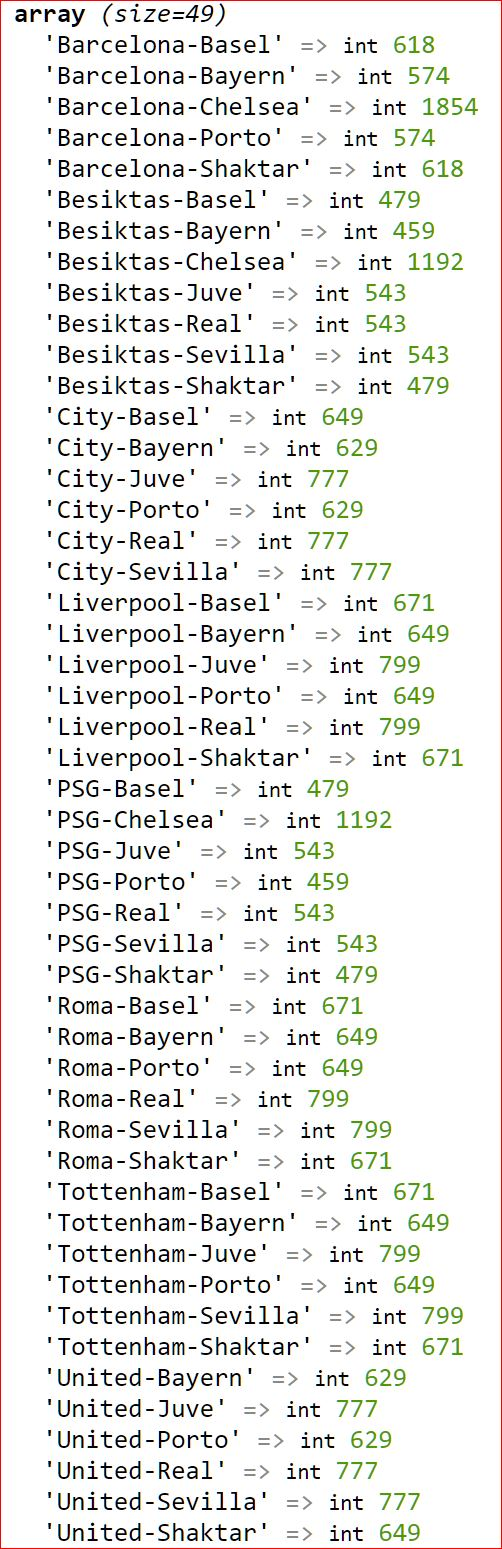I calculate the associated percentage for each pair (example for Liverpool-Real it’s `799 out of 4238=18.85%`) and, after half an hour spent choosing a color scheme, I put everything in the matrix: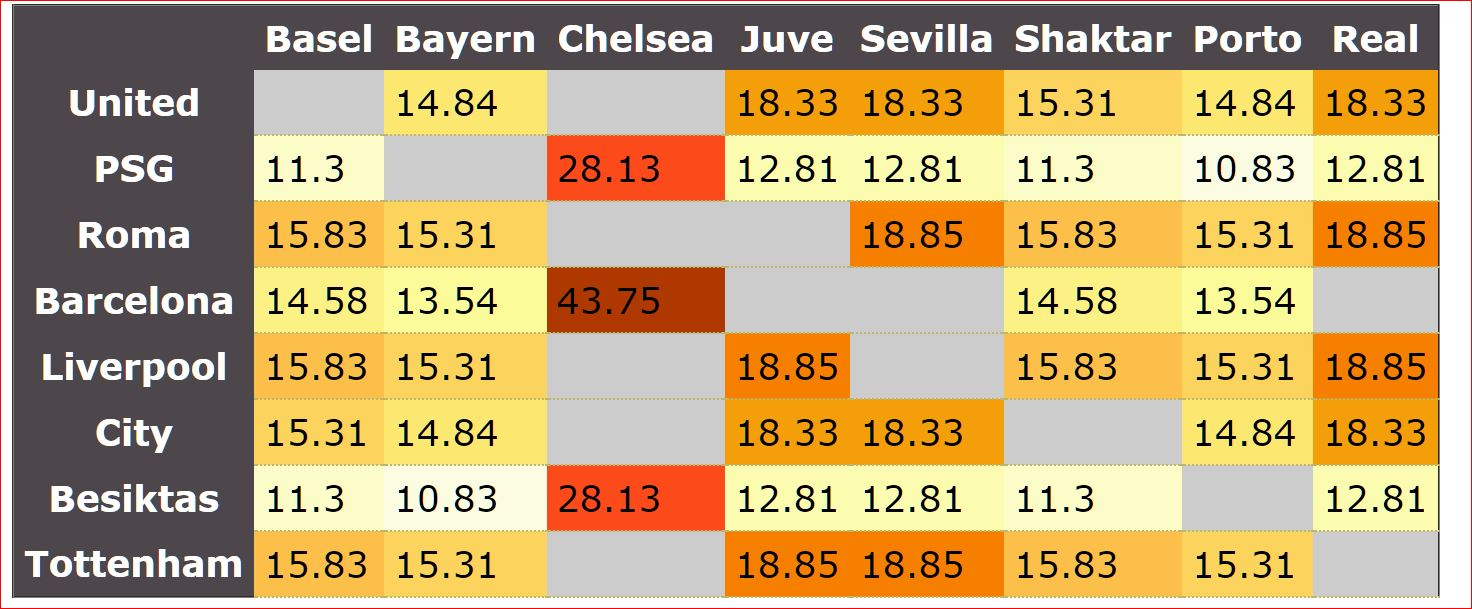Then I realize that the numbers are slightly different from the ones circulated on social media: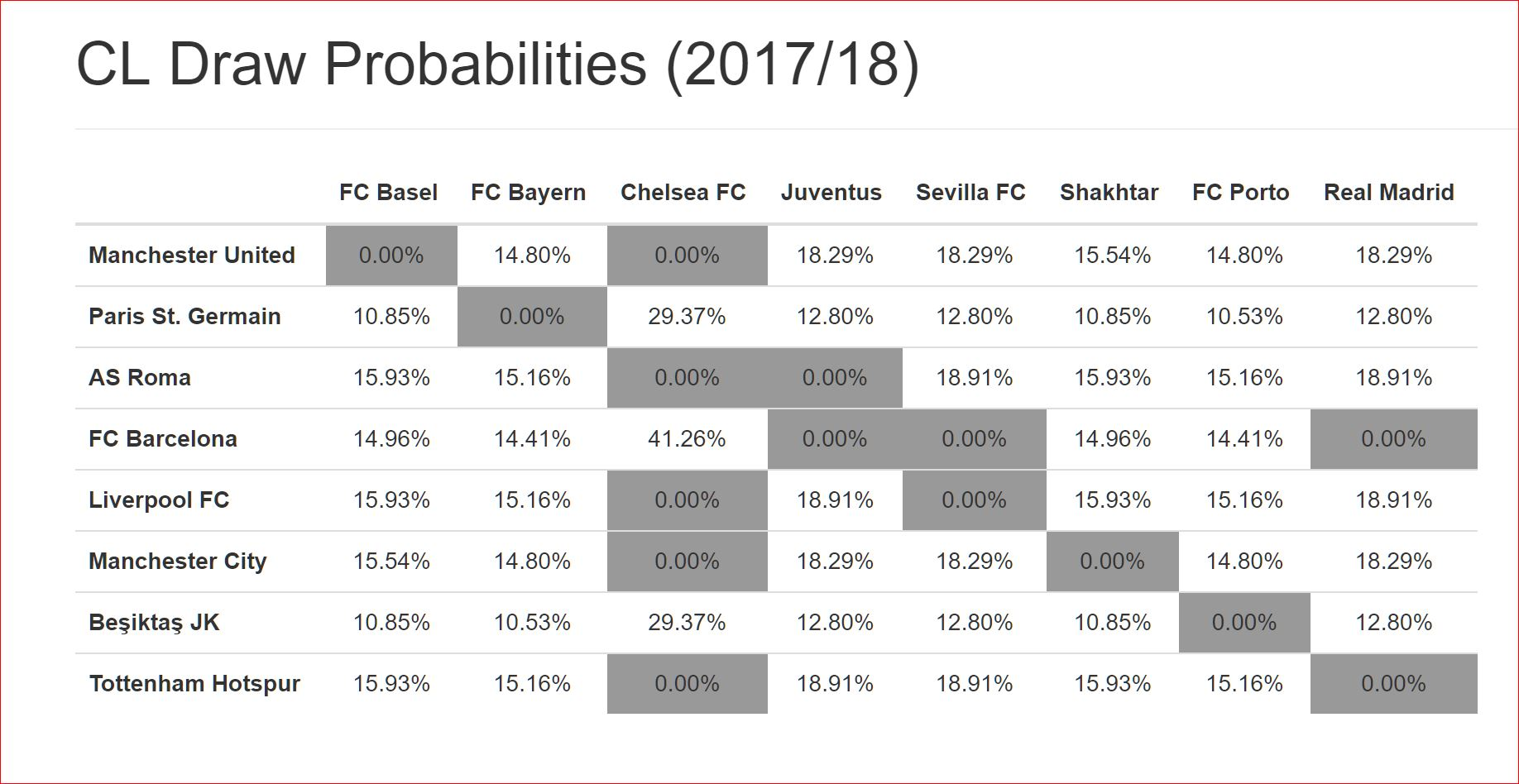## Try 2: the entire weekend

I get a very nice explanation on Twitter from the author of the tool above:

Then I start to realize that my approach was incorrect.
In fact, my numbers were only valid if the draw process consisted of a single step – somebody picking up a random number from 1 to 4238 and then showing up the 8 pairings behind that number.
But in fact, the draw process consist of 8 steps or 8 events, each one depending on the previous one. We speak in this case of conditional probabilities, which are represented using a probability tree. The probability tree for a subset of 6 teams looks like this: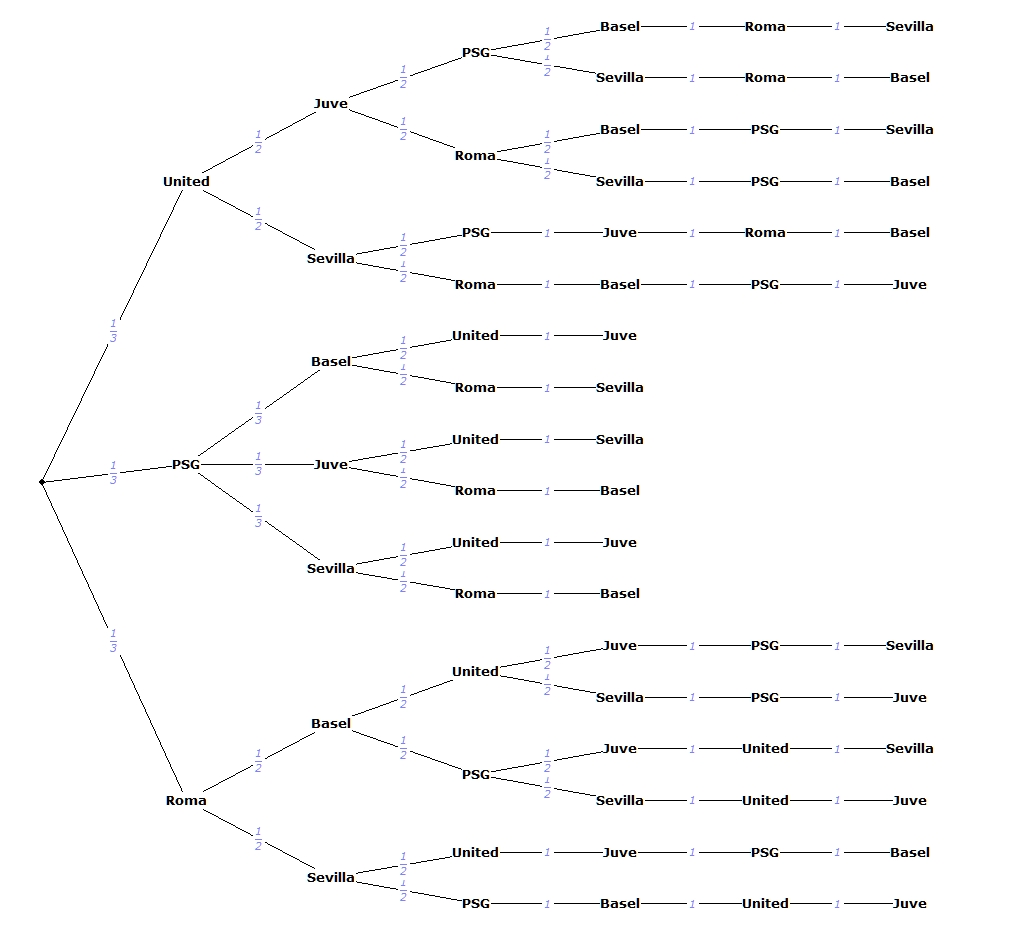And indeed, the tree simulates the real draw process and reveals the same numbers as the ‘official’ ones: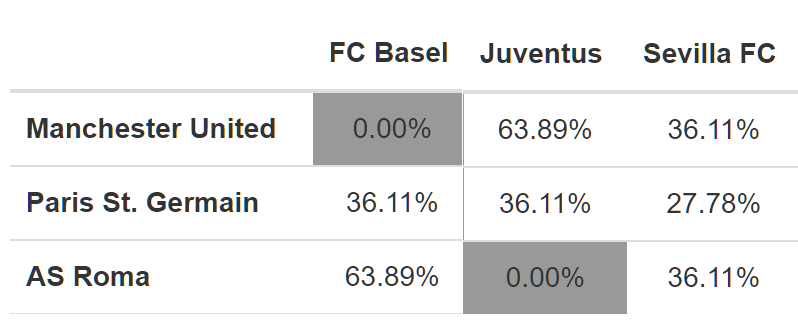Since the draw is in less than 12 hours, I have no time to make another script that generates the full tree (that would also be too big to put in a picture). But I trust the numbers from https://eminga.github.io/cldraw/ are correct 🙂

## Random things #8

Written on 18 March 2015, 10:28pm

Tagged with: , , , , ,

## A/B vs Multivariate Testing

A/B testing: two versions (A and B) are compared, which are identical except for one variation that might affect a user’s behavior. Total number of variations: 2. More
Multivariate testing: multiple variables are modified for testing a hypothesis. The goal of multivariate testing is to determine which combination of variations performs the best out of all of the possible combinations. `[Total # of Variations] = [# of Variations on Element A] X [# of Variations on Element B] ...` More

## Permutations, Arrangements, Combinations

Given a set of n elements (ex – for n=3, the set is A, B, C)
Permutations: each ordered set of n elements `P(n) = n! `
In our example with n=3, P(3)=3!=6: АВС, АСВ, ВАС, ВСА, САВ, СВА
Arrangements: each ordered set of k elements `A(n,k) = n! / (n-k)!`
In our example with n=3, ordered pairs of 2, A(3,2)=3!/(3-2)!=6: AB, BA, AC, CA, BC, CB
Combinations: each unordered set of k elements `C(n,k) = n! / k! (n-k)!`
In our example with n=3, un-ordered pairs of 2, C(3,2)=3!/2!*1!=3: AB, AC, BC
And the relationship between P, A, C: `C=A/P`
Remember that for the permutations you don’t need a k! More